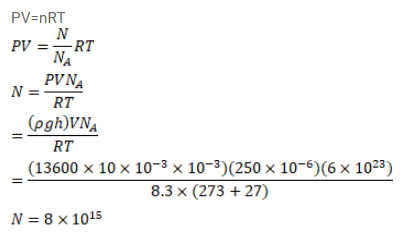# Use R=8.3 J/mol-K wherever required.Question:

Use $R=8.3 \mathrm{~J} / \mathrm{mol}-\mathrm{K}$ wherever required.

An electric bulb of volume 250 cc was sealed during manufacturing at a pressure of $10^{-3} \mathrm{~mm}$ of mercury at $27^{\circ} \mathrm{C}$. Compute the number of air molecules contained in the bulb. Avogadro constant $=6^{\times} 10^{23}$ per $\mathrm{mol}$, density of mercury $=13600 \mathrm{~kg} / \mathrm{m}^{3}$ and $\mathrm{g}=10 \mathrm{~m} / \mathrm{s}^{2}$.

Solution: